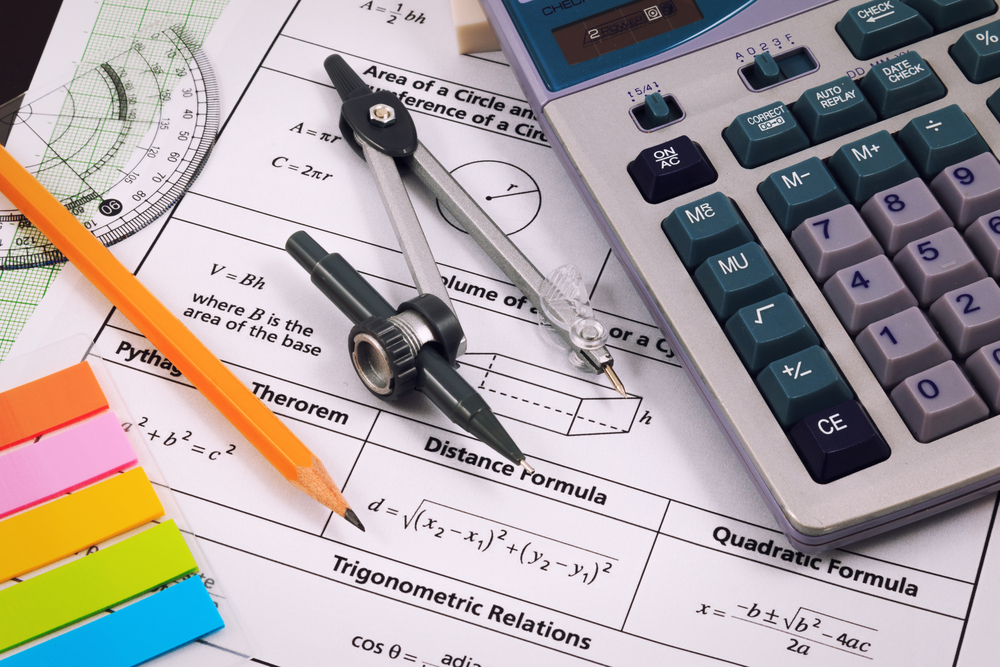# What do I need to learn to achieve a Grade 6 in Maths?

While many pupils are aiming to achieve the highest grades possible in GCSE Maths, a growing number of students are asking how to achieve a grade 6 in Maths.

This should not be seen as being a lack of motivation, there are a large, and growing, number of post 16 courses that have a requirement of achieving a grade 6 or higher at GCSE Maths. Quite frequently, the students who are in this situation want to focus on other subjects that have a more direct use in their chosen field.## Which topics do I need to learn to get Grade 6 in maths?

When we want to ask, ‘what do we need to learn to achieve a grade 6 in Maths?’, we run into the same problem I discussed in a previous post about levels. It is virtually impossible to say with any certainty that ‘this topic is a grade 6 topic’, the following are my thoughts on what is likely to come up on the first half of the paper.

### Firstly, look at the first half of the Higher paper.

The way that the new Maths exam papers are structured is that the first 50% of the paper is targeted at pupils who are capable of grades 4,5 and 6 and the second half is aimed at 7, 8 and 9. What we can take from this is that if you are trying to achieve grade 6, you can almost ignore the second half of the paper and focus your time on getting the first half spot on.

### Which topics will come up?

We can get a good idea of what will come up by looking into the GCSE specification. If you look at the programme of study, some of the topics are written in bold. As this means that the topic is higher tier only, we can say that anything which is not in bold will be both foundation and higher topics so will appear, either directly or indirectly, on the paper.

As we know that grade 6 is the first higher specific level, we can look at the higher topics from the specification and think what are the ‘simpler’ topics that are likely to come up in the first half of the higher paper.

Number: Calculating in terms of π. While this does appear at the foundation level, it is more likely to come up at Higher Tier when you are dealing with sectors and compound shapes.

Algebra: Parallel gradients (just remember that parallel lines have the same gradient) and finding the equation of a straight line, solving quadratics by factorising including factorising equations of the form ax2 + bx + c, creating and solving simultaneous equations and finally the nth term of a quadratic and non -linear sequences

Ratio and Proportion: Using the Pressure, Force and Area formulas, note that these are frequently tied in with shape questions.

Statistics: Cumulative frequency and box plots. This is a lovely little topic, if you practice these on past papers, it will probably be exactly the same style of question.

## How to revise for GCSE maths grade 6

I wrote a post recently on the methods of revising for GCSE Maths, one of which was using past papers. Most past papers have the same questions at the end of the foundation paper as at the start of the higher tier.

One of the tips I will add to that post is that if you are trying to achieve a grade 6, it could be worth looking at the second half of the foundation papers to see if there are any additional questions that don’t appear on the higher tier.